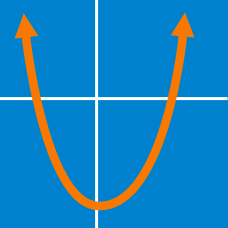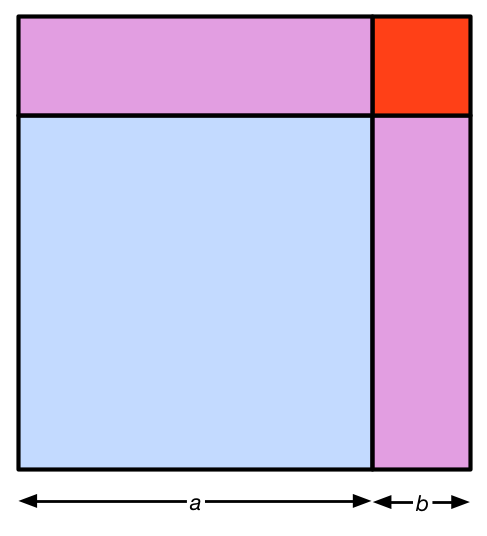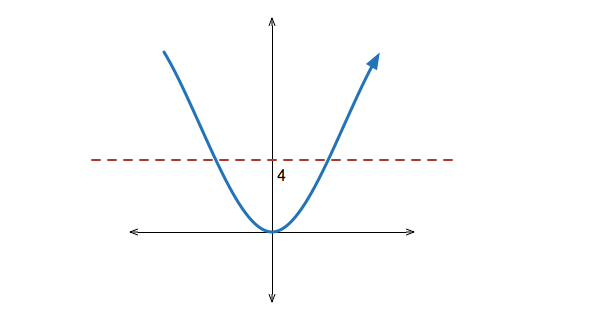Algebra

# Quadratic Equations: Level 2 ChallengesThe image above illustrates which identity?

When the square of a certain positive integer is reduced by 38, we get the value 83. What is the integer?

Suppose that 6 is one of the roots of quadratic equation $x^2-2x+a=0$. What is the other root?

\begin{aligned} x(y+z)&=&39\\ y(x+z)&=&60\\ z(x+y)&=&63 \\ x^2+y^2+z^2&=& \ ? \end{aligned}

See Part 2 and Part 3.What are the solutions to the equation

$x^2 = 4 ?$

×